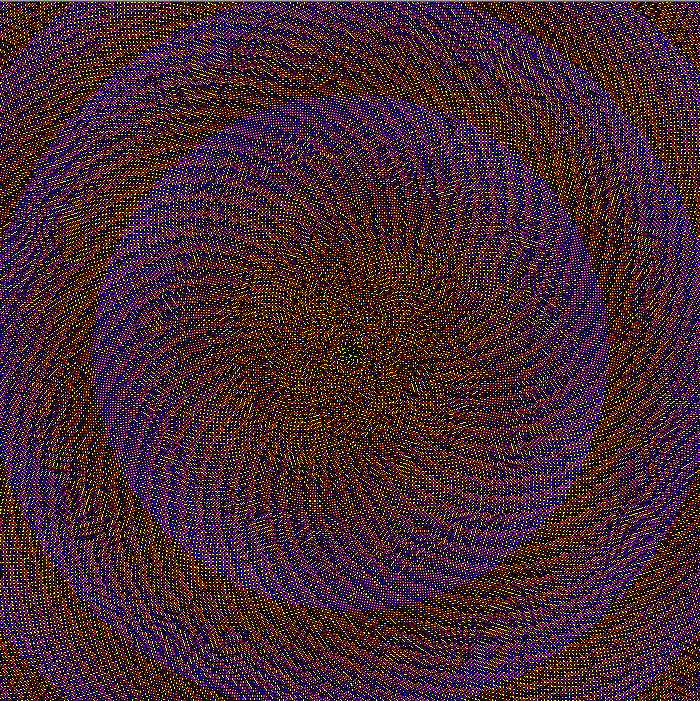Categories

# Sunflower Layout in Processing

For a while, I’ve been wanting to make something that explores color in photo sets. Reading Beautiful Visualization (Amazon), I came across the perfect way to arrange the elements. It imitates the layout of the sunflower seeds, “the most efficient and visually mesmerizing way of packing small elements into a large circle”.

I decided to try the layout in Processing and to keep it simple, by following an easy rule for creating color. Start with black (the absence of color) and add red, then when red was saturated add blue, and finally green until we reach white. Because there is no yellow in the pattern, I used yellow for the background.

I’m not entirely happy with the very centre (I think this is a consequence of having to convert between double and float) but it’s pretty cool:Using a different strategy for changing color (essentially generating every even numbered RGB value) with smaller radius and spacing, I made the image below.I love this layout!

I use Java in Eclipse with the core.jar library. You can use this code in the Processing Editor with some small modifications.

## Code

```import processing.core.PApplet;

public class SunflowerSeeds extends PApplet {

private static final int radius = 10;
private static final int scale = 7;

private static final double goldenangle = Math.PI * (3 - Math.sqrt(5));

private static final int wh = 400;

public void setup() {
size(wh, wh);
background(255, 255, 0);
noLoop();
}

public void draw() {
int r = 0;
int g = 0;
int b = 0;

int n = 0;

double a = 0;

while (g < 255) {
if (r < 255) {
r++;
}
else if (b < 255) {
b++;
}
else {
g++;
}

double h = Math.sqrt(n)*scale;
double x = wh/2 + Math.sin(a) * h;
double y = wh/2 + Math.cos(a) * h;

stroke(0);
fill(r, g, b);

a+=goldenangle;
n++;
}
}
}
```

## Code for Animated Version

```import processing.core.PApplet;

public class SunflowerAnimated extends PApplet{

private static final int radius = 10;
private static final int scale = 7;

private static final double goldenangle = Math.PI * (3 - Math.sqrt(5));

private static final int wh = 400;

private int r = 0;
private int g = 0;
private int b = 0;

private int n = 0;
private double a = 0;

public void setup() {
size(wh, wh);
background(255, 255, 0);
}

public void draw() {
if (g >= 255) {
noLoop();
return;
}
else if (r < 255) {
r++;
}
else if (b < 255) {
b++;
}
else {
g++;
}

double h = Math.sqrt(n)*scale;
double x = wh/2 + Math.sin(a) * h;
double y = wh/2 + Math.cos(a) * h;
a+=goldenangle;
stroke(0);
fill(r, g, b);

n++;
}
}```

## 3 replies on “Sunflower Layout in Processing”Thealtman01says:

that’s beautiful. i just learned about Processing today. after quitting python because i couldn’t get from writing code to drawing lines fast enough, this is an especially welcome toolkit.Â

[…] found the perfect layout for this, the sunflower layout, and then did nothing about it for… a long time. I claim the craziness of work and life. […]

[…] Back in 2011. I wanted to be able to visualize a series of photos. I found the perfect layout (the sunflower layout) and thenâ€¦ did nothing with it. Later I returned to it and discovered that extracting the […]